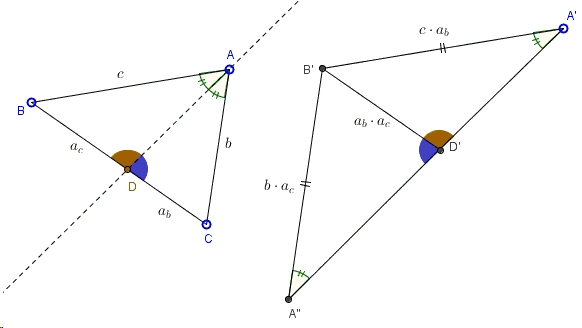# Property of Internal Angle Bisector - Hubert Shutrick's PWW

Let $AD$ be an angle bisector in $\Delta ABC$,Then $\displaystyle\frac{b}{c}=\frac{a_{b}}{a_{c}}$, where $b=AC$, $c=AB$, $a_{b}=CD$, $a_{c}=BD$.

(The applet below illustrates a proof by Hubert Shutrick. Points $A$, $B$, $C$, $A'$ are draggable.

### Proof

This proof without words is due to Hubert Shutrick.This is another example showing how the product identities that you get algebraically from similar triangles can be illustrated. The method applies in several additional situations.

(There are several theorems that are proved by similar technique.)### Angle Bisector